﻿Average Directional Movement Rating

# Average Directional Movement Rating

Average Directional Movement Rating Technical Indicator (Adxr) helps to determine if there is a price trend. It consists of five lines: ADX, ADXR, AD, +DI, -DI.Market Signals

The simplest trading method based on the system of directional movement implies comparison of two direction indicators: the 14-period +DI one and the 14-period -DI. To do this, one either puts the charts of indicators one on top of the other, or +DI is subtracted from -DI. It should buy when +DI is higher than -DI, and sell when +DI sinks lower than -DI.

Also there are “a rule of points of extremum" to eliminate false signals and decrease the numbers of deals. It is used to eliminate false signals and decrease the number of deals. According to the principle of points of extremum, the "point of extremum" is the point when +DI and -DI cross each other. If +DI raises higher than -DI, this point will be the maximum price of the day when they cross. If +DI is lower than -DI, this point will be the minimum price of the day they cross.

The point of extremum is used then as the market entry level. Thus, after the signal to buy (+DI is higher than -DI) one must wait till the price has exceeded the point of extremum, and only then buy. However, if the price fails to exceed the level of the point of extremum, one should retain the short position.Calculation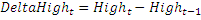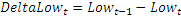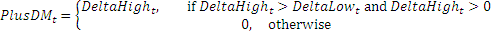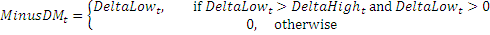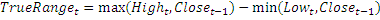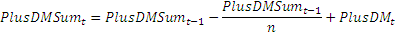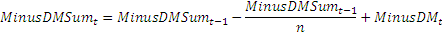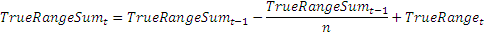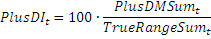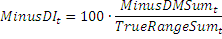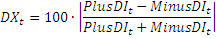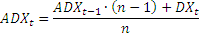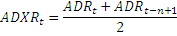Chart Example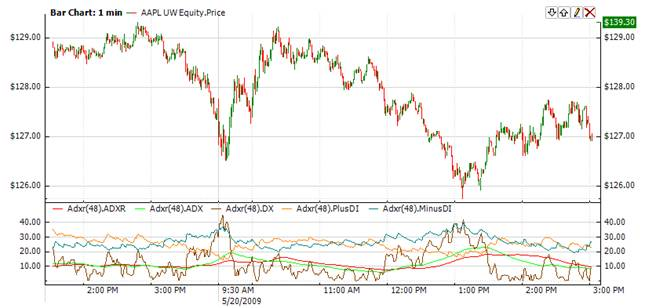Implementation and Usage

To initialize Adxr indicator use the following constructors:

Adxr - this constructor sets default value of 14 for period.

Adxr(Int32) - sets period of the indicator

Use

DX

PlusDI

MinusDI

properies to get current valueExample
C#
``` 1// Create new instance
3
4// Number of stored values
6
9
10// Get indicator value